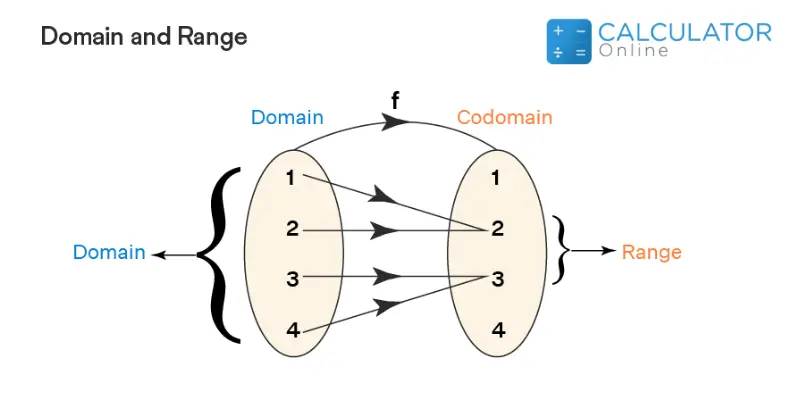Uh Oh! It seems you’re using an Ad blocker!

We always struggled to serve you with the best online calculations, thus, there's a humble request to either disable the AD blocker or go with premium plans to use the AD-Free version for calculators.

Or# Domain and Range Calculator

Write the function in the designated field and the tool will display the domain and range of the function.

Equation:

Equation Preview:

Table of Content

Get the Widget!

Add this calculator to your site and lets users to perform easy calculations.

Feedback

How easy was it to use our calculator? Did you face any problem, tell us!

The online domain and range calculator with steps finds domain and range for a function in a couple of clicks. Examines the range in which the domain of a certain mathematical function exists. Not only this, but you will also get results in proper set interval notations.

## What Is the Domain?

Particular set of values that help to define a function after they are put in it by our domain calculator.

## What Is the Range?

The set of values that the function yields after the domain values are put in it.

### Example:

Consider the figure below:In the following figure:

• D is not concerned with any of the range entities, so it is not considered as the domain of the function
• Likewise, the number 2 is not linked with any domain element, yielding it as an odd man out for range

In actual, calculating domain and range of the function will let you investigate the behaviour

### How to Find Domain and Range of a Function?

Go through the example below to better understand how to find the domain of a function along with its range!

#### Statement:

Find domain and range of the graph function given as under:

$$y=\dfrac{x+3}{10-x}$$

#### Solution:

##### Domain:

First, look for the value of x that will make the denominator zero. In our case, it is 10, such that;

$$10-x = 10-10 = 0$$

So 10 is the number that undefines the whole expression. This is why it is not included in the domain.

##### Range:

Solving for x:

$$y=\dfrac{x+3}{10-x}$$

$$y\left(10-x\right)=x+3$$

$$10y-xy=x+3$$

$$-xy-x=3-10y$$

$$-x\left(y+1\right)$$

$$-x=\dfrac{3-10y}{\left(y+1\right)}$$

$$x=\dfrac{10y-3}{-\left(y+1\right)}$$

Now if you put value of y as -1, it will again make the denominator as zero such that:

$$x=\dfrac{10y-3}{-\left(\left(-1\right)+1\right)}$$

### How Does Domain and Range Calculator Function Work?

Want to calculate domain and range of functions through our domain finder? Follow the guide below!

Input:

• Enter your function and hit calculate to find results

Output:

• Domain and range of the function

### Is 7 a Domain or Range?

7 means y=7, and it indicates a straight line equation. Coming to the point, its domain is all real numbers and range is 7 only. For further verification, you may put the expression in the online domain and range calculator with steps to nullify your doubts.

## References:

From the source of Wikipedia: Domain of a function, Natural domain, Set theoretical notions

From the source of Khan Academy: Domain and range from graph, Intervals